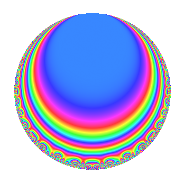# Properties

 Label 804.2.g.bLevel 804 Weight 2 Character orbit 804.g Analytic conductor 6.420 Analytic rank 0 Dimension 4 CM No Inner twists 4

# Related objects

## Newspace parameters

 Level: $$N$$ = $$804 = 2^{2} \cdot 3 \cdot 67$$ Weight: $$k$$ = $$2$$ Character orbit: $$[\chi]$$ = 804.g (of order $$2$$ and degree $$1$$)

## Newform invariants

 Self dual: No Analytic conductor: $$6.41997232251$$ Analytic rank: $$0$$ Dimension: $$4$$ Coefficient field: $$\Q(\sqrt{-2}, \sqrt{-5})$$ Coefficient ring: $$\Z[a_1, \ldots, a_{5}]$$ Coefficient ring index: $$1$$ Sato-Tate group: $\mathrm{SU}(2)[C_{2}]$

## $q$-expansion

Coefficients of the $$q$$-expansion are expressed in terms of a basis $$1,\beta_1,\beta_2,\beta_3$$ for the coefficient ring described below. We also show the integral $$q$$-expansion of the trace form.

 $$f(q)$$ $$=$$ $$q -\beta_{1} q^{3} + ( 2 \beta_{1} - \beta_{2} ) q^{5} + \beta_{2} q^{7} + ( 2 + \beta_{3} ) q^{9} +O(q^{10})$$ $$q -\beta_{1} q^{3} + ( 2 \beta_{1} - \beta_{2} ) q^{5} + \beta_{2} q^{7} + ( 2 + \beta_{3} ) q^{9} + ( 2 \beta_{1} - \beta_{2} ) q^{11} -3 \beta_{2} q^{13} + ( -5 - \beta_{3} ) q^{15} + 3 \beta_{3} q^{17} -3 q^{19} + ( 1 - \beta_{3} ) q^{21} + 3 \beta_{3} q^{23} + 5 q^{25} + ( -\beta_{1} - 3 \beta_{2} ) q^{27} -\beta_{3} q^{29} + 3 \beta_{2} q^{31} + ( -5 - \beta_{3} ) q^{33} + 2 \beta_{3} q^{35} + 5 q^{37} + ( -3 + 3 \beta_{3} ) q^{39} + ( 8 \beta_{1} - 4 \beta_{2} ) q^{41} -8 \beta_{2} q^{43} + ( 4 \beta_{1} + 3 \beta_{2} ) q^{45} -\beta_{3} q^{47} + 5 q^{49} + ( 3 \beta_{1} - 9 \beta_{2} ) q^{51} + 10 q^{55} + 3 \beta_{1} q^{57} -\beta_{3} q^{59} + 2 \beta_{2} q^{61} + ( -2 \beta_{1} + 3 \beta_{2} ) q^{63} -6 \beta_{3} q^{65} + ( -7 + 3 \beta_{2} ) q^{67} + ( 3 \beta_{1} - 9 \beta_{2} ) q^{69} -6 \beta_{3} q^{71} + 5 q^{73} -5 \beta_{1} q^{75} + 2 \beta_{3} q^{77} + 10 \beta_{2} q^{79} + ( -1 + 4 \beta_{3} ) q^{81} + 2 \beta_{3} q^{83} + 15 \beta_{2} q^{85} + ( -\beta_{1} + 3 \beta_{2} ) q^{87} + \beta_{3} q^{89} + 6 q^{91} + ( 3 - 3 \beta_{3} ) q^{93} + ( -6 \beta_{1} + 3 \beta_{2} ) q^{95} -6 \beta_{2} q^{97} + ( 4 \beta_{1} + 3 \beta_{2} ) q^{99} +O(q^{100})$$ $$\operatorname{Tr}(f)(q)$$ $$=$$ $$4q + 8q^{9} + O(q^{10})$$ $$4q + 8q^{9} - 20q^{15} - 12q^{19} + 4q^{21} + 20q^{25} - 20q^{33} + 20q^{37} - 12q^{39} + 20q^{49} + 40q^{55} - 28q^{67} + 20q^{73} - 4q^{81} + 24q^{91} + 12q^{93} + O(q^{100})$$

Basis of coefficient ring in terms of a root $$\nu$$ of $$x^{4} - 4 x^{2} + 9$$:

 $$\beta_{0}$$ $$=$$ $$1$$ $$\beta_{1}$$ $$=$$ $$\nu$$ $$\beta_{2}$$ $$=$$ $$($$$$\nu^{3} - \nu$$$$)/3$$ $$\beta_{3}$$ $$=$$ $$\nu^{2} - 2$$
 $$1$$ $$=$$ $$\beta_0$$ $$\nu$$ $$=$$ $$\beta_{1}$$ $$\nu^{2}$$ $$=$$ $$\beta_{3} + 2$$ $$\nu^{3}$$ $$=$$ $$3 \beta_{2} + \beta_{1}$$

## Character Values

We give the values of $$\chi$$ on generators for $$\left(\mathbb{Z}/804\mathbb{Z}\right)^\times$$.

 $$n$$ $$269$$ $$337$$ $$403$$ $$\chi(n)$$ $$-1$$ $$-1$$ $$1$$

## Embeddings

For each embedding $$\iota_m$$ of the coefficient field, the values $$\iota_m(a_n)$$ are shown below.

For more information on an embedded modular form you can click on its label.

Label $$\iota_m(\nu)$$ $$a_{2}$$ $$a_{3}$$ $$a_{4}$$ $$a_{5}$$ $$a_{6}$$ $$a_{7}$$ $$a_{8}$$ $$a_{9}$$ $$a_{10}$$
401.1
 1.58114 + 0.707107i 1.58114 − 0.707107i −1.58114 + 0.707107i −1.58114 − 0.707107i
0 −1.58114 0.707107i 0 3.16228 0 1.41421i 0 2.00000 + 2.23607i 0
401.2 0 −1.58114 + 0.707107i 0 3.16228 0 1.41421i 0 2.00000 2.23607i 0
401.3 0 1.58114 0.707107i 0 −3.16228 0 1.41421i 0 2.00000 2.23607i 0
401.4 0 1.58114 + 0.707107i 0 −3.16228 0 1.41421i 0 2.00000 + 2.23607i 0
 $$n$$: e.g. 2-40 or 990-1000 Significant digits: Format: Complex embeddings Normalized embeddings Satake parameters Satake angles

## Inner twists

Char. orbit Parity Mult. Self Twist Proved
1.a Even 1 trivial yes
3.b Odd 1 yes
67.b Odd 1 yes
201.d Even 1 yes

## Hecke kernels

This newform can be constructed as the kernel of the linear operator $$T_{5}^{2} - 10$$ acting on $$S_{2}^{\mathrm{new}}(804, [\chi])$$.